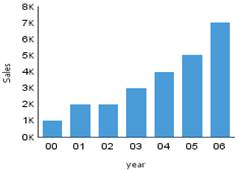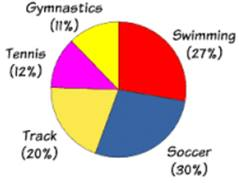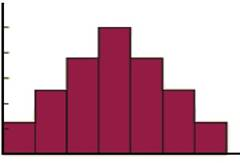1. /
2. CBSE
3. /
4. Class 08
5. /
6. Introduction to Graphs class...

# Introduction to Graphs class 8 Notes Mathematics### myCBSEguide App

Download the app to get CBSE Sample Papers 2023-24, NCERT Solutions (Revised), Most Important Questions, Previous Year Question Bank, Mock Tests, and Detailed Notes.

Introduction to Graphs class 8 Notes Mathematics in PDF are available for free download in myCBSEguide mobile app. The best app for CBSE students now provides Introduction to Graphs class 8 Notes Mathematics latest chapter wise notes for quick preparation of CBSE exams and school-based annual examinations. Class 8 Mathematics notes on chapter 15 Introduction to Graphs Mathematics are also available for download in CBSE Guide website.

## Class 8 Mathematics notes Chapter 15 Introduction to Graphs

Download CBSE class 8th revision notes for chapter 15 Introduction to Graphs in PDF format for free. Download revision notes for Introduction to Graphs class 8 Notes and score high in exams. These are the Introduction to Graphs class 8 Notes prepared by team of expert teachers. The revision notes help you revise the whole chapter 15 in minutes. Revision notes in exam days is one of the best tips recommended by teachers during exam days.

## CBSE Class 8 Revision Notes Science Introduction to Graphs

• Graphical presentation of data is easier to understand.
(i) A bar graph is used to show comparison among categories.
(ii) A pie graph is used to compare parts of a whole.
(iii) A Histogram is a bar graph that shows data in intervals.
• A  line graph displays data that changes continuously over periods of time.
• A line graph which is a whole unbroken line is called a  linear graph.
• For fixing a point on the graph sheet we need, x-coordinate and y-coordinate.
• The relation between dependent variable and independent variable is shown through a graph.
• A Bar Graph: A pictorial representation of numerical data in the form of bars (rectangles) of uniform width with equal spacing. The length (or height) of each bar represents the given number.• A Pie Graph: A pie graph is used to compare parts of a whole. The various observations or components are represented by the sectors of the circle.• A Histogram: Histogram is a type of bar diagram, where the class intervals are shown on the horizontal axis and the heights of the bars (rectangles) show the frequency of the class interval, but there is no gap between the bars as there is no gap between the class intervals.• Linear Graph: A line graph in which all the line segments form a part of a single line.
• Coordinates: A point in Cartesian plane is represented by an ordered pair of numbers.
• Ordered Pair: A pair of numbers written in specified order.

## CBSE Class 8 Revision Notes and Key Points

Introduction to Graphs class 8 Notes Mathematics. CBSE quick revision note for class-8 Mathematics, Chemistry, Math’s, Biology and other subject are very helpful to revise the whole syllabus during exam days. The revision notes covers all important formulas and concepts given in the chapter. Even if you wish to have an overview of a chapter, quick revision notes are here to do if for you. These notes will certainly save your time during stressful exam days.

To download Introduction to Graphs class 8 Notes Mathematics, sample paper for class 8 Mathematics, Social Science, Science, Mathematics; do check myCBSEguide app or website. myCBSEguide provides sample papers with solution, test papers for chapter-wise practice, NCERT solutions, NCERT Exemplar solutions, quick revision notes for ready reference, CBSE guess papers and CBSE important question papers. Sample Paper all are made available through the best app for CBSE students and myCBSEguide website.### Test Generator

Create question paper PDF and online tests with your own name & logo in minutes.### myCBSEguide

Question Bank, Mock Tests, Exam Papers, NCERT Solutions, Sample Papers, Notes